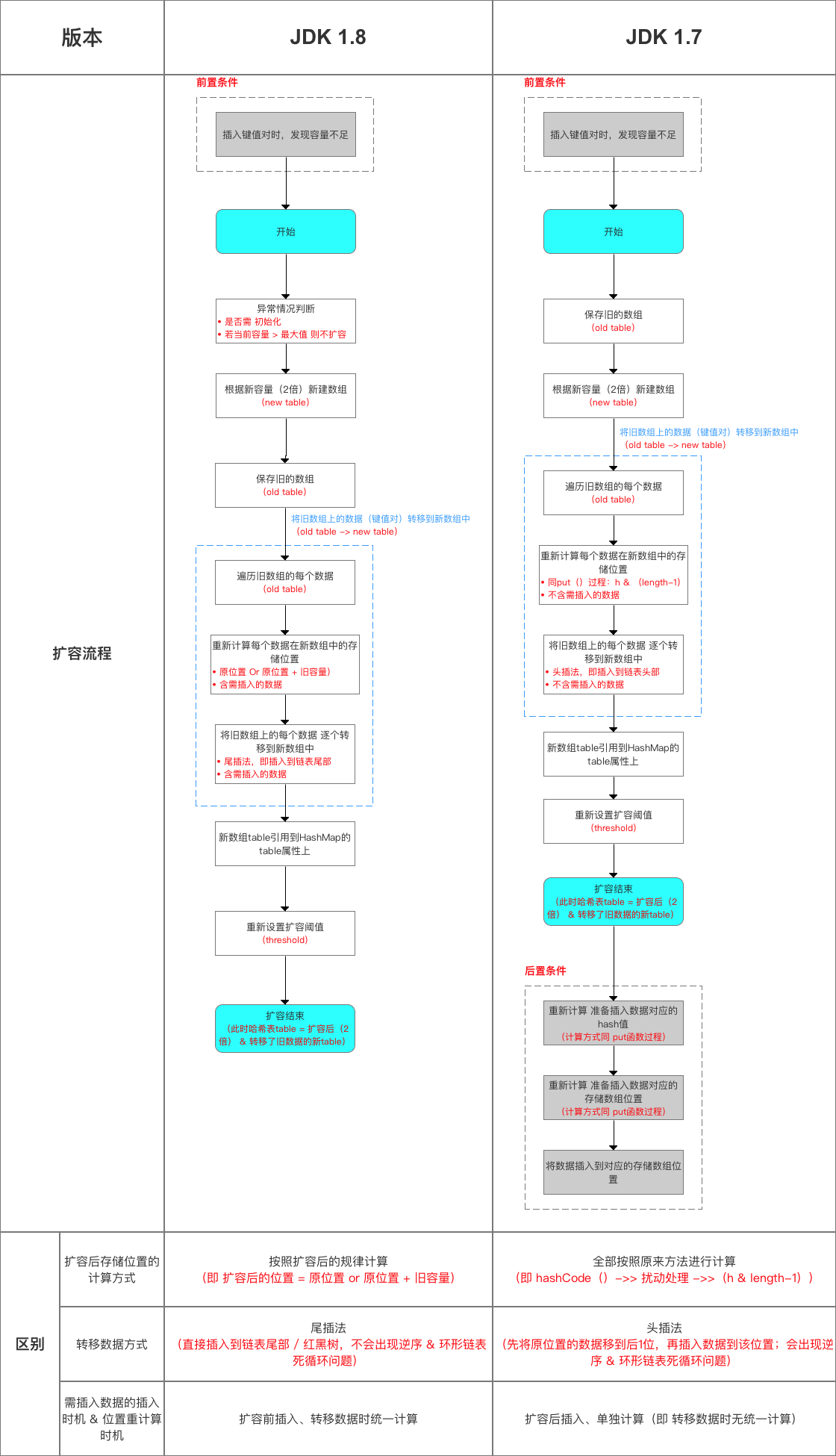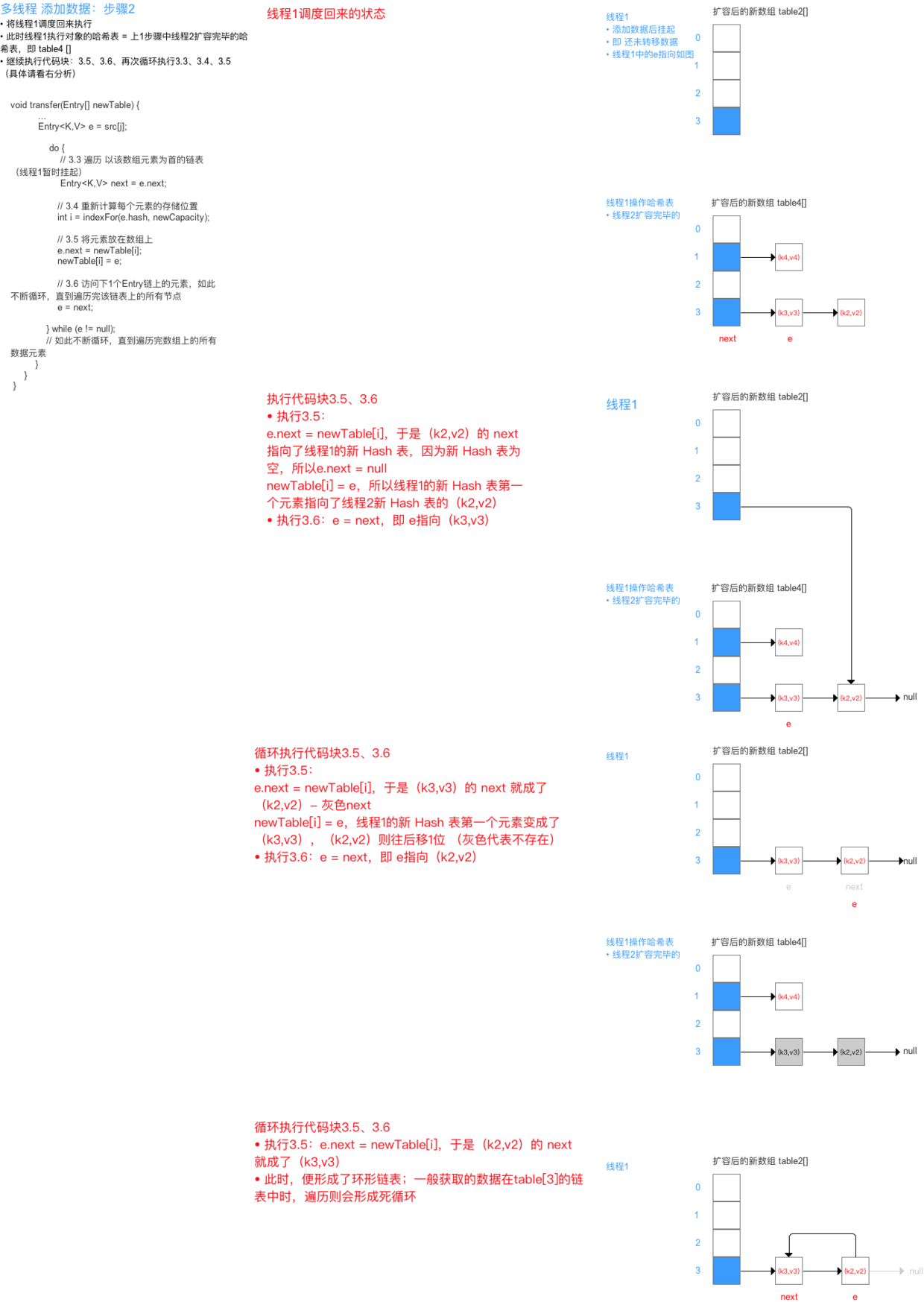1 概述

HashMap是基于哈希表实现的,每一个元素是一个key-value对,其内部通过单链表解决冲突问题,容量不足(超过了阀值)时,同样会自动增长.

HashMap是非线程安全的,只适用于单线程环境,多线程环境可以采用并发包下的concurrentHashMap

HashMap 实现了Serializable接口，因此它支持序列化，实现了Cloneable接口，能被克隆

HashMap是基于哈希表的Map接口的非同步实现.此实现提供所有可选的映射操作,并允许使用null值和null键.此类不保证映射的顺序,特别是它不保证该顺序恒久不变.

Java8中又对此类底层实现进行了优化，比如引入了红黑树的结构以解决哈希碰撞

2 HashMap的数据结构

HashMap实际上是一个"链表散列"的数据结构,即数组和链表的结合体.HashMap的主结构类似于一个数组,添加值时通过key确定储存位置.3 三大集合与迭代子

HashMap使用三大集合和三种迭代子来轮询其Key、Value和Entry对象

public class HashMapExam {
public static void main(String[] args) {
Map<Integer, String> map = new HashMap<>(16);
for (int i = 0; i < 15; i++) {
map.put(i, new String(new char[]{(char) ('A'+ i)}));
}

System.out.println("======keySet=======");
Set<Integer> set = map.keySet();
Iterator<Integer> iterator = set.iterator();
while (iterator.hasNext()) {
System.out.println(iterator.next());
}

System.out.println("======values=======");
Collection<String> values = map.values();
Iterator<String> stringIterator=values.iterator();
while (stringIterator.hasNext()) {
System.out.println(stringIterator.next());
}

System.out.println("======entrySet=======");
for (Map.Entry<Integer, String> entry : map.entrySet()) {
System.out.println(entry);
}
}
}

4 源码分析
    //默认的初始容量16,且实际容量是2的整数幂
static final int DEFAULT_INITIAL_CAPACITY = 1 << 4;

//最大容量(传入容量过大将被这个值替换)
static final int MAXIMUM_CAPACITY = 1 << 30;

// 默认加载因子为0.75(当表达到3/4满时,才会再散列),这个因子在时间和空间代价之间达到了平衡.更高的因子可以降低表所需的空间,但是会增加查找代价,而查找是最频繁操作
static final float DEFAULT_LOAD_FACTOR = 0.75f;

//桶的树化阈值：即 链表转成红黑树的阈值，在存储数据时，当链表长度 >= 8时，则将链表转换成红黑树
static final int TREEIFY_THRESHOLD = 8;
// 桶的链表还原阈值：即 红黑树转为链表的阈值，当在扩容（resize（））时（HashMap的数据存储位置会重新计算），在重新计算存储位置后，当原有的红黑树内数量 <= 6时，则将 红黑树转换成链表
static final int UNTREEIFY_THRESHOLD = 6;
//最小树形化容量阈值：即 当哈希表中的容量 > 该值时，才允许树形化链表 （即 将链表 转换成红黑树）


    // 为了避免扩容/树形化选择的冲突，这个值不能小于 4 * TREEIFY_THRESHOLD
// 小于该值时使用的是扩容哦!!!
static final int MIN_TREEIFY_CAPACITY = 64;

// 存储数据的Node数组,长度是2的幂.
// HashMap采用链表法解决冲突，每一个Node本质上是一个单向链表
//HashMap底层存储的数据结构,是一个Node数组.上面得知Node类为元素维护了一个单向链表.至此,HashMap存储的数据结构也就很清晰了:维护了一个数组,每个数组又维护了一个单向链表.之所以这么设计,考虑到遇到哈希冲突的时候,同index的value值就用单向链表来维护
//与 JDK 1.7 的对比（Entry类），仅仅只是换了名字
transient Node<K,V>[] table;

// HashMap的底层数组中已用槽的数量
transient int size;
// HashMap的阈值，用于判断是否需要调整HashMap的容量（threshold = 容量*加载因子）
int threshold;

// 负载因子实际大小

// HashMap被改变的次数
transient int modCount;

// 指定“容量大小”和“加载因子”的构造函数,是最基础的构造函数
public HashMap(int initialCapacity, float loadFactor) {
if (initialCapacity < 0)
throw new IllegalArgumentException("Illegal initial capacity: " +
initialCapacity);
// HashMap的最大容量只能是MAXIMUM_CAPACITY
if (initialCapacity > MAXIMUM_CAPACITY)
initialCapacity = MAXIMUM_CAPACITY;
//负载因子须大于0
throw new IllegalArgumentException("Illegal load factor: " +
// 设置"负载因子"
// 设置"HashMap阈值",当HashMap中存储数据的数量达到threshold时,就需将HashMap的容量加倍
this.threshold = tableSizeFor(initialCapacity);
}

• 上面的tableSizeFor有何用?
tableSizeFor方法保证函数返回值是大于等于给定参数initialCapacity最小的2的幂次方的数值
    static final int tableSizeFor(int cap) {
int n = cap - 1;
n |= n >>> 1;
n |= n >>> 2;
n |= n >>> 4;
n |= n >>> 8;
n |= n >>> 16;
return (n < 0) ? 1 : (n >= MAXIMUM_CAPACITY) ? MAXIMUM_CAPACITY : n + 1;
}


a |= b 等同于 a = a|b

• int n = cap - 1

• n |= n >>> 1

n >>> 1 : n无符号右移1位,即n二进制最高位的1右移一位

n | (n >>> 1) 导致 n二进制的高2位值为1

• n |= n >>> 2

n继续无符号右移2位

n | (n >>> 2) 导致n二进制表示的高3~4位经过运算值均为1

• n |= n >>> 4

n继续无符号右移4位

n | (n >>> 4) 导致n二进制表示的高5~8位经过运算值均为1

• n |= n >>> 8

n继续无符号右移8位

n | (n >>> 8) 导致n二进制表示的高9~16位经过运算值均为14.1为什么cap要保持为2的幂次方？HashMap中存储数据table的index是由key的Hash值决定的.index = e.hash % newCap4.2Node类
static class Node<K,V> implements Map.Entry<K,V> {
final int hash;
final K key;
V value;
Node<K,V> next;

Node(int hash, K key, V value, Node<K,V> next) {
this.hash = hash;
this.key = key;
this.value = value;
this.next = next;
}

public final K getKey()        { return key; }
public final V getValue()      { return value; }
public final String toString() { return key + "=" + value; }

public final int hashCode() {
return Objects.hashCode(key) ^ Objects.hashCode(value);
}

public final V setValue(V newValue) {
V oldValue = value;
value = newValue;
return oldValue;
}

public final boolean equals(Object o) {
if (o == this)
return true;
if (o instanceof Map.Entry) {
Map.Entry<?,?> e = (Map.Entry<?,?>)o;
if (Objects.equals(key, e.getKey()) &&
Objects.equals(value, e.getValue()))
return true;
}
return false;
}
}



Node<K,V> 类是HashMap中的静态内部类,实现Map.Entry<K,V>接口.定义了key键、value值、next节点,也就是说元素之间构成了单向链表.

4.3 TreeNode
static final class TreeNode<K,V> extends LinkedHashMap.Entry<K,V> {
TreeNode<K,V> parent;  // red-black tree links
TreeNode<K,V> left;
TreeNode<K,V> right;
TreeNode<K,V> prev;    // needed to unlink next upon deletion
boolean red;
TreeNode(int hash, K key, V val, Node<K,V> next) {}

// 返回当前节点的根节点
final TreeNode<K,V> root() {
for (TreeNode<K,V> r = this, p;;) {
if ((p = r.parent) == null)
return r;
r = p;
}
}
}


4.4 hash方法

Java 8中的散列值优化函数key.hashCode()函数调用的是key键值类型自带的哈希函数，返回int型散列值“与”操作的结果就是散列值的高位全部归零，只保留低位值，用来做数组下标访问

2进制表示是00000000 00000000 00001111index的运算规则是

e.hash & (newCap - 1)


newCap是2的幂,所以newCap - 1的高位全0

4.5 Put方法①.判断键值对数组table[i]是否为空或为null，否则执行resize()进行扩容

②.根据键值key计算hash值得到插入的数组索引i，如果table[i]==null，直接新建节点添加，转向⑥，如果table[i]不为空，转向③

③.判断table[i]的首个元素是否和key一样，如果相同直接覆盖value，否则转向④，这里的相同指的是hashCode以及equals

④.判断table[i] 是否为treeNode，即table[i] 是否是红黑树，如果是红黑树，则直接在树中插入键值对，否则转向⑤

⑤.遍历table[i]，判断链表长度是否大于8，大于8的话把链表转换为红黑树，在红黑树中执行插入操作，否则进行链表的插入操作；遍历过程中若发现key已经存在直接覆盖value即可

⑥.插入成功后，判断实际存在的键值对数量size是否超多了最大容量threshold，如果超过，执行resize()扩容

 public V put(K key, V value) {
// 对key的hashCode()做hash
return putVal(hash(key), key, value, false, true);
}

final V putVal(int hash, K key, V value, boolean onlyIfAbsent, boolean evict) {
Node<K,V>[] tab; Node<K,V> p; int n, i;
// 步骤① tab为空则调用resize()初始化创建
if ((tab = table) == null || (n = tab.length) == 0)
n = (tab = resize()).length;
// 步骤② 计算index,并对null做处理
//tab[i = (n - 1) & hash对应下标的第一个节点
if ((p = tab[i = (n - 1) & hash]) == null)
// 无哈希冲突的情况下,将value直接封装为Node并赋值
tab[i] = newNode(hash, key, value, null);
else {
Node<K,V> e; K k;
// 步骤③ 节点的key相同,直接覆盖节点
if (p.hash == hash && ((k = p.key) == key || (key != null && key.equals(k))))
e = p;
// 步骤④ 判断该链为红黑树
else if (p instanceof TreeNode)
// p是红黑树类型，则调用putTreeVal方式赋值
e = ((TreeNode<K,V>)p).putTreeVal(this, tab, hash, key, value);
// 步骤⑤ p非红黑树类型,该链为链表
else {
// index 相同的情况下
for (int binCount = 0; ; ++binCount) {
if ((e = p.next) == null) {
// 如果p的next为空,将新的value值添加至链表后面
p.next = newNode(hash, key, value, null);
if (binCount >= TREEIFY_THRESHOLD - 1)
// 如果链表长度大于8,链表转化为红黑树,执行插入
treeifyBin(tab, hash);
break;
}
// key相同则跳出循环
if (e.hash == hash &&  ((k = e.key) == key || (key != null && key.equals(k))))
break;
//就是移动指针方便继续取 p.next

p = e;
}
}
if (e != null) { // existing mapping for key
V oldValue = e.value;
//根据规则选择是否覆盖value
if (!onlyIfAbsent || oldValue == null)
e.value = value;
afterNodeAccess(e);
return oldValue;
}
}
++modCount;
// 步骤⑥:超过最大容量,就扩容
if (++size > threshold)
// size大于加载因子,扩容
resize();
afterNodeInsertion(evict);
return null;
}


4.6 resize/**
* 该函数有2种使用情况：1.初始化哈希表 2.当前数组容量过小，需扩容
*/
final Node<K,V>[] resize() {
Node<K,V>[] oldTab = table;
int oldCap = (oldTab == null) ? 0 : oldTab.length;
int oldThr = threshold;
int newCap, newThr = 0;

// 针对情况2：若扩容前的数组容量超过最大值，则不再扩充
if (oldCap > 0) {
if (oldCap >= MAXIMUM_CAPACITY) {
threshold = Integer.MAX_VALUE;
return oldTab;
}
// 针对情况2：若无超过最大值，就扩充为原来的2倍
else if ((newCap = oldCap << 1) < MAXIMUM_CAPACITY &&
oldCap >= DEFAULT_INITIAL_CAPACITY)
//newCap设置为oldCap的2倍并小于MAXIMUM_CAPACITY，且大于默认值, 新的threshold增加为原来的2倍
newThr = oldThr << 1; // double threshold
}

// 针对情况1：初始化哈希表（采用指定 or 默认值）
else if (oldThr > 0) // initial capacity was placed in threshold
// threshold>0, 将threshold设置为newCap,所以要用tableSizeFor方法保证threshold是2的幂次方
newCap = oldThr;
else {               // zero initial threshold signifies using defaults
// 默认初始化
newCap = DEFAULT_INITIAL_CAPACITY;
}

// 计算新的resize上限
if (newThr == 0) {
// newThr为0，newThr = newCap * 0.75
float ft = (float)newCap * loadFactor;
newThr = (newCap < MAXIMUM_CAPACITY && ft < (float)MAXIMUM_CAPACITY ?
(int)ft : Integer.MAX_VALUE);
}
threshold = newThr;
@SuppressWarnings({"rawtypes","unchecked"})
// 新生成一个table数组
Node<K,V>[] newTab = (Node<K,V>[])new Node[newCap];
table = newTab;
if (oldTab != null) {
// oldTab 复制到 newTab
for (int j = 0; j < oldCap; ++j) {
Node<K,V> e;
if ((e = oldTab[j]) != null) {
oldTab[j] = null;
if (e.next == null)
// 链表只有一个节点，直接赋值
//为什么要重新Hash呢？因为长度扩大以后，Hash的规则也随之改变。
newTab[e.hash & (newCap - 1)] = e;
else if (e instanceof TreeNode)
// e为红黑树的情况
((TreeNode<K,V>)e).split(this, newTab, j, oldCap);
else { // preserve order链表优化重hash的代码块
Node<K,V> loHead = null, loTail = null;
Node<K,V> hiHead = null, hiTail = null;
Node<K,V> next;
do {
next = e.next;
// 原索引
if ((e.hash & oldCap) == 0) {
if (loTail == null)
else
loTail.next = e;
loTail = e;
}
// 原索引 + oldCap
else {
if (hiTail == null)
else
hiTail.next = e;
hiTail = e;
}
} while ((e = next) != null);
// 原索引放到bucket里
if (loTail != null) {
loTail.next = null;
}
// 原索引+oldCap放到bucket里
if (hiTail != null) {
hiTail.next = null;
}
}
}
}
}
return newTab;
}4.7 remove方法

remove(key) 方法 和 remove(key, value) 方法都是通过调用removeNode的方法来实现删除元素的

 final Node<K,V> removeNode(int hash, Object key, Object value,
boolean matchValue, boolean movable) {
Node<K,V>[] tab; Node<K,V> p; int n, index;
if ((tab = table) != null && (n = tab.length) > 0 &&
(p = tab[index = (n - 1) & hash]) != null) {
Node<K,V> node = null, e; K k; V v;
if (p.hash == hash &&
((k = p.key) == key || (key != null && key.equals(k))))
// index 元素只有一个元素
node = p;
else if ((e = p.next) != null) {
if (p instanceof TreeNode)
// index处是一个红黑树
node = ((TreeNode<K,V>)p).getTreeNode(hash, key);
else {
// index处是一个链表，遍历链表返回node
do {
if (e.hash == hash &&
((k = e.key) == key ||
(key != null && key.equals(k)))) {
node = e;
break;
}
p = e;
} while ((e = e.next) != null);
}
}
// 分不同情形删除节点
if (node != null && (!matchValue || (v = node.value) == value ||
(value != null && value.equals(v)))) {
if (node instanceof TreeNode)
((TreeNode<K,V>)node).removeTreeNode(this, tab, movable);
else if (node == p)
tab[index] = node.next;
else
p.next = node.next;
++modCount;
--size;
afterNodeRemoval(node);
return node;
}
}
return null;
}


4.8 get
/**
* 函数原型
* 作用：根据键key，向HashMap获取对应的值
*/
map.get(key)；

/**
* 源码分析
*/
public V get(Object key) {
Node<K,V> e;
// 1\. 计算需获取数据的hash值
// 2\. 通过getNode（）获取所查询的数据 ->>分析1
// 3\. 获取后，判断数据是否为空
return (e = getNode(hash(key), key)) == null ? null : e.value;
}

/**
* 分析1：getNode(hash(key), key))
*/
final Node<K,V> getNode(int hash, Object key) {
Node<K,V>[] tab; Node<K,V> first, e; int n; K k;

// 1\. 计算存放在数组table中的位置
if ((tab = table) != null && (n = tab.length) > 0 &&
(first = tab[(n - 1) & hash]) != null) {

// 4\. 通过该函数，依次在数组、红黑树、链表中查找（通过equals（）判断）
// a. 先在数组中找，若存在，则直接返回
if (first.hash == hash && // always check first node
((k = first.key) == key || (key != null && key.equals(k))))
return first;

// b. 若数组中没有，则到红黑树中寻找
if ((e = first.next) != null) {
// 在树中get
if (first instanceof TreeNode)
return ((TreeNode<K,V>)first).getTreeNode(hash, key);

// c. 若红黑树中也没有，则通过遍历，到链表中寻找
do {
if (e.hash == hash &&
((k = e.key) == key || (key != null && key.equals(k))))
return e;
} while ((e = e.next) != null);
}
}
return null;
}


/**
* 源码分析：resize(2 * table.length)
* 作用：当容量不足时（容量 > 阈值），则扩容（扩到2倍）
*/
void resize(int newCapacity) {

// 1\. 保存旧数组（old table）
Entry[] oldTable = table;

// 2\. 保存旧容量（old capacity ），即数组长度
int oldCapacity = oldTable.length;

// 3\. 若旧容量已经是系统默认最大容量了，那么将阈值设置成整型的最大值，退出
if (oldCapacity == MAXIMUM_CAPACITY) {
threshold = Integer.MAX_VALUE;
return;
}

// 4\. 根据新容量（2倍容量）新建1个数组，即新table
Entry[] newTable = new Entry[newCapacity];

// 5\. （重点分析）将旧数组上的数据（键值对）转移到新table中，从而完成扩容 ->>分析1.1
transfer(newTable);

// 6\. 新数组table引用到HashMap的table属性上
table = newTable;

// 7\. 重新设置阈值
}

/**
* 分析1.1：transfer(newTable);
* 作用：将旧数组上的数据（键值对）转移到新table中，从而完成扩容
* 过程：按旧链表的正序遍历链表、在新链表的头部依次插入
*/
void transfer(Entry[] newTable) {
// 1\. src引用了旧数组
Entry[] src = table;

// 2\. 获取新数组的大小 = 获取新容量大小
int newCapacity = newTable.length;

// 3\. 通过遍历 旧数组，将旧数组上的数据（键值对）转移到新数组中
for (int j = 0; j < src.length; j++) {
// 3.1 取得旧数组的每个元素
Entry<K,V> e = src[j];
if (e != null) {
// 3.2 释放旧数组的对象引用（for循环后，旧数组不再引用任何对象）
src[j] = null;

do {
// 3.3 遍历 以该数组元素为首 的链表
// 注：转移链表时，因是单链表，故要保存下1个结点，否则转移后链表会断开
Entry<K,V> next = e.next;
// 3.3 重新计算每个元素的存储位置
int i = indexFor(e.hash, newCapacity);
// 3.4 将元素放在数组上：采用单链表的头插入方式 = 在链表头上存放数据 = 将数组位置的原有数据放在后1个指针、将需放入的数据放到数组位置中
// 即 扩容后，可能出现逆序：按旧链表的正序遍历链表、在新链表的头部依次插入
e.next = newTable[i];
newTable[i] = e;
// 访问下1个Entry链上的元素，如此不断循环，直到遍历完该链表上的所有节点
e = next;
} while (e != null);
// 如此不断循环，直到遍历完数组上的所有数据元素
}
}
}4.9 getOrDefault

getOrDefault() 方法获取指定 key 对应对 value，如果找不到 key ，则返回设置的默认值。5 单线程rehash6 多线程并发下的rehashe.next = newTable = null
newTable = e = key(5)
e = next = key(9)7 Fast-fail 产生原因

HashIterator() {
expectedModCount = modCount;
if (size > 0) { // advance to first entry
Entry[] t = table;
while (index < t.length && (next = t[index++]) == null)
;
}
}